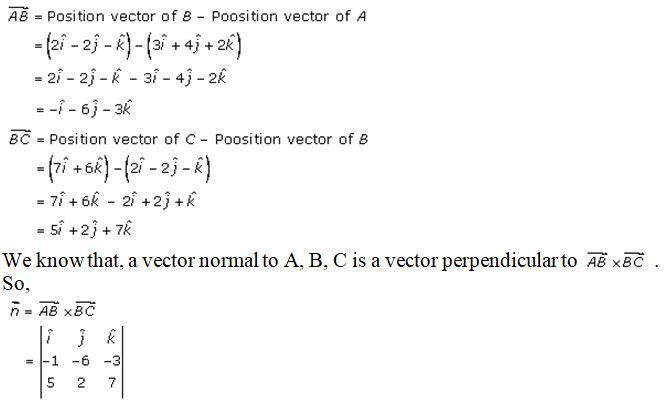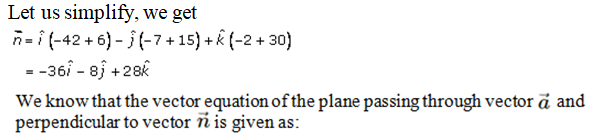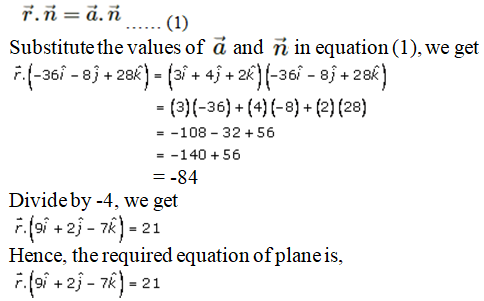# RD Sharma Solutions Class 12 The Plane Exercise 29.5

RD Sharma Solutions for Class 12 Maths Chapter 29 The Plane Exercise 29.5, is given here for students to easily access the solutions. These solutions are completely based on the exam-oriented approach to help students score well in their board examination. Practising these questions will ensure that they can easily excel in their final exams. Here, the RD Sharma Solutions to this exercise are provided in pdf format, which can be downloaded easily from the links given below.

## Download PDF of RD Sharma Solutions For Class 12 Maths Chapter 29 Exercise 5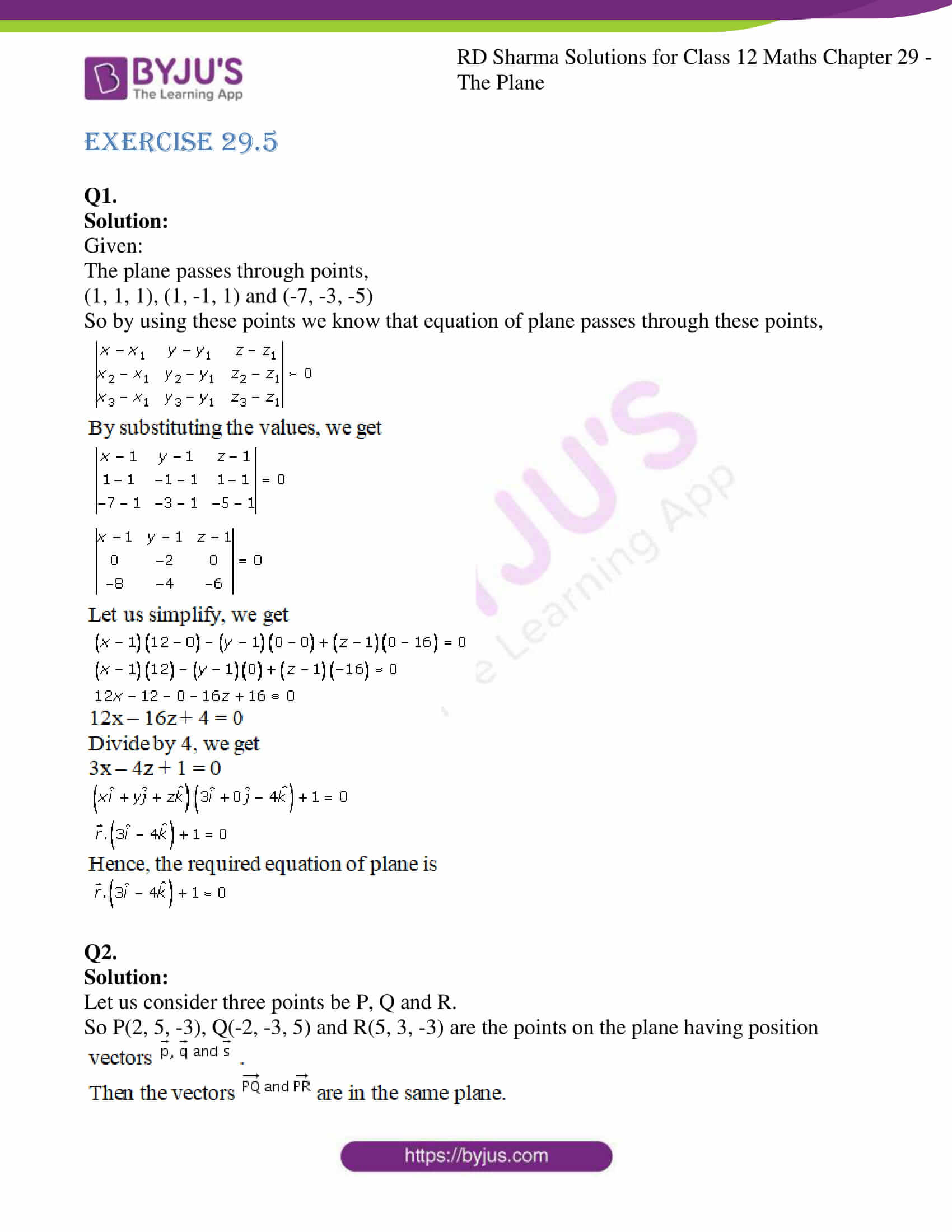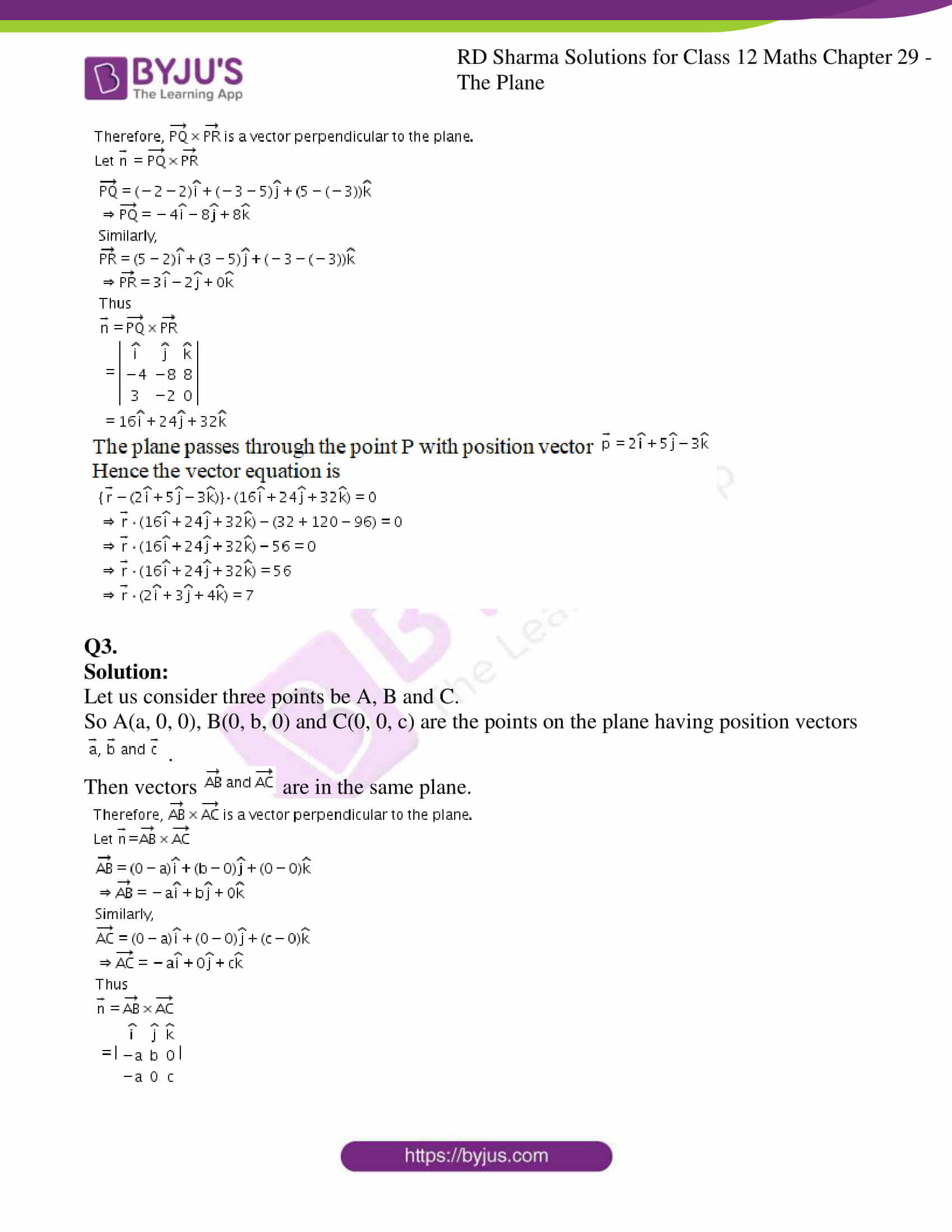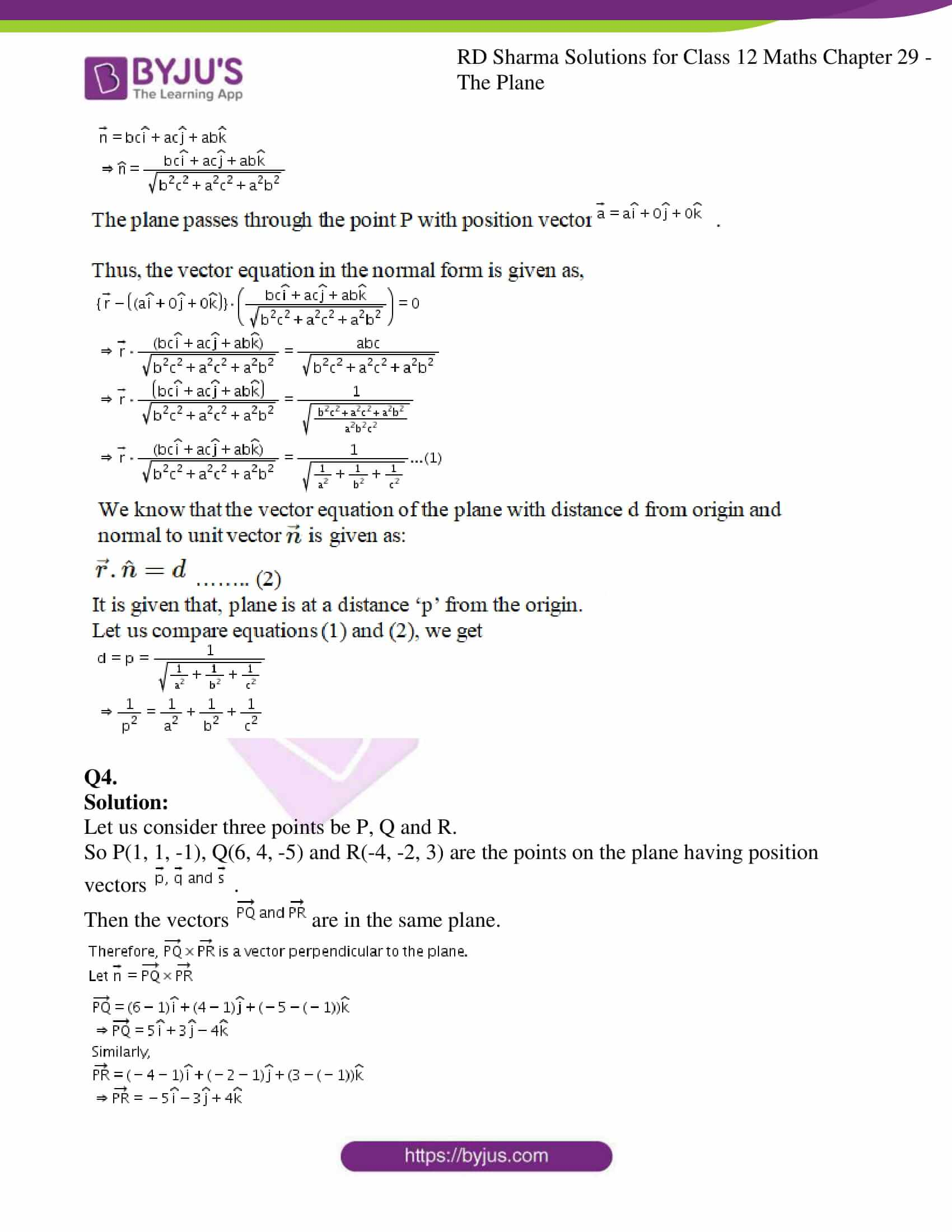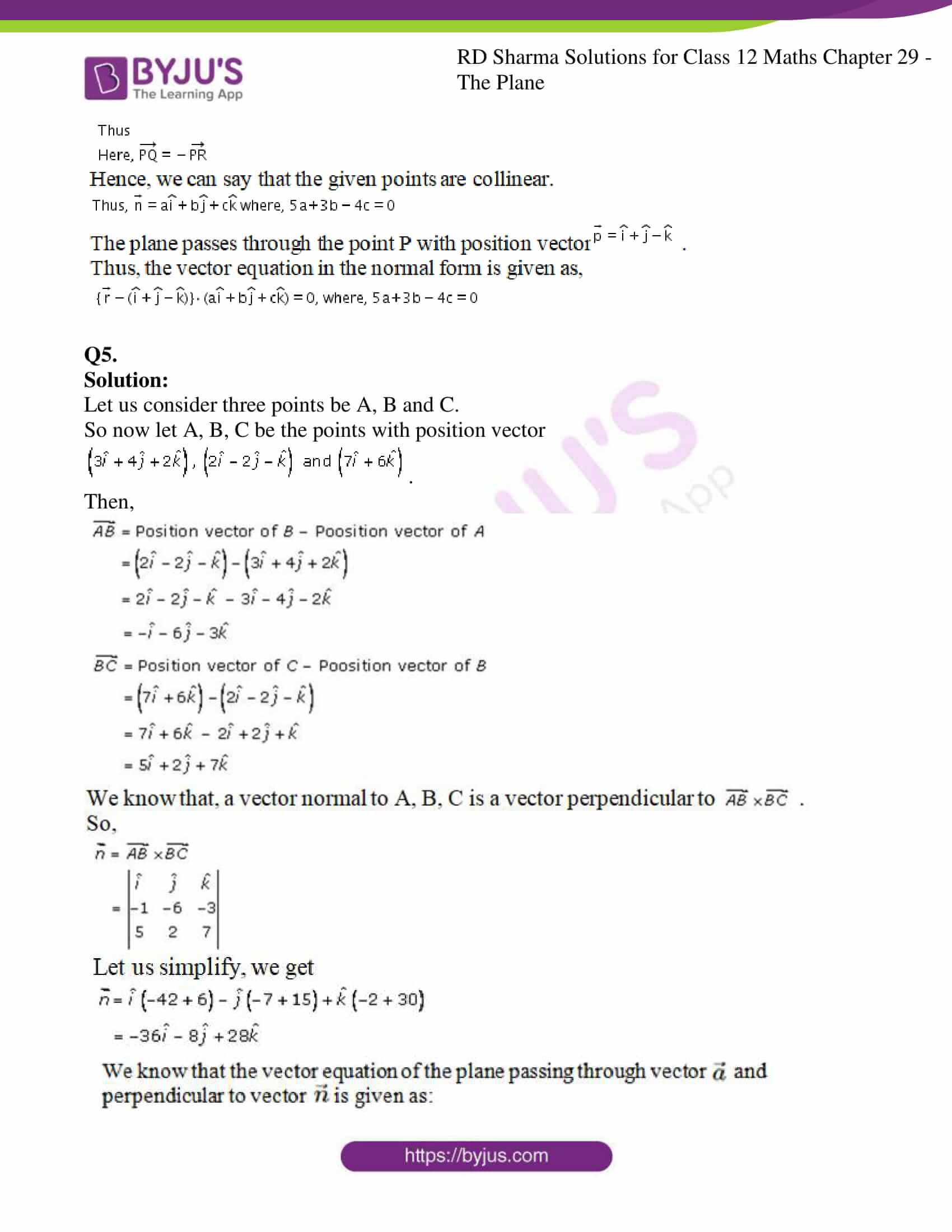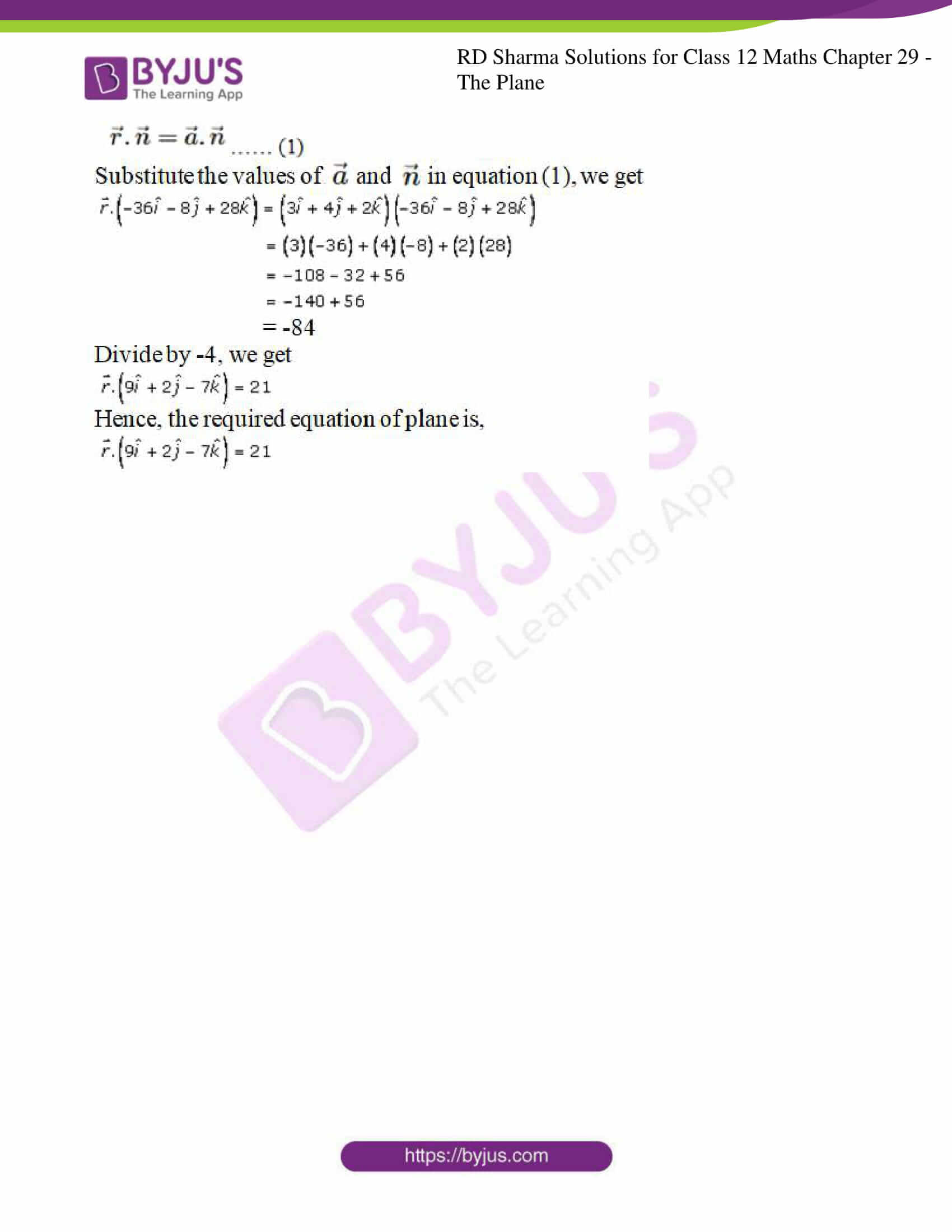### Access RD Sharma Solutions For Class 12 Maths Chapter 29 Exercise 5

EXERCISE 29.5

Q1.

Solution:

Given:

The plane passes through points,

(1, 1, 1), (1, -1, 1) and (-7, -3, -5)

So by using these points we know that equation of plane passes through these points,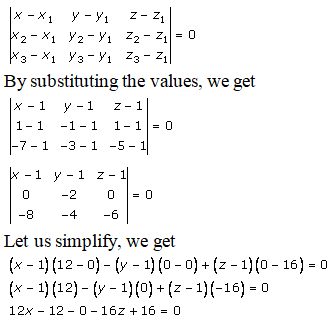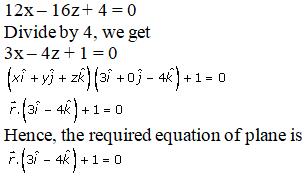Q2.

Solution:

Let us consider three points be P, Q and R.

So P(2, 5, -3), Q(-2, -3, 5) and R(5, 3, -3) are the points on the plane having position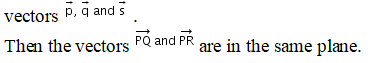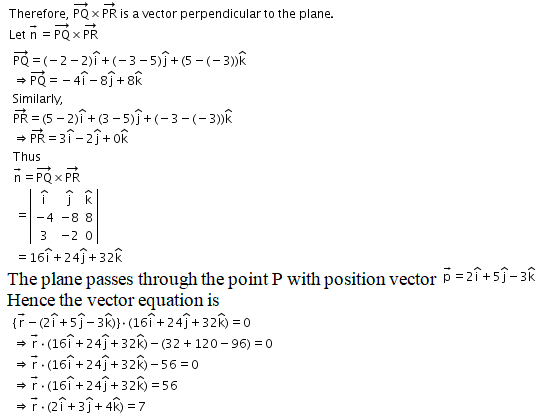Q3.

Solution:

Let us consider three points be A, B and C.

So A(a, 0, 0), B(0, b, 0) and C(0, 0, c) are the points on the plane having position vectors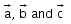.

Then vectors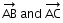are in the same plane.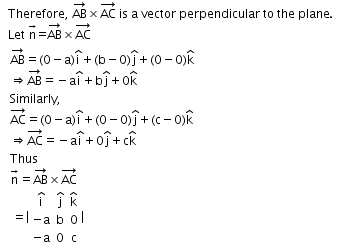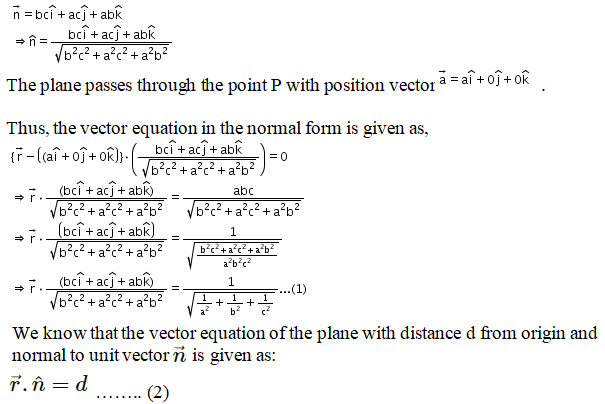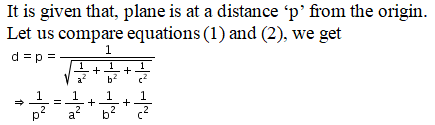Q4.

Solution:

Let us consider three points be P, Q and R.

So P(1, 1, -1), Q(6, 4, -5) and R(-4, -2, 3) are the points on the plane having position vectors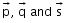.

Then the vectors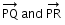are in the same plane.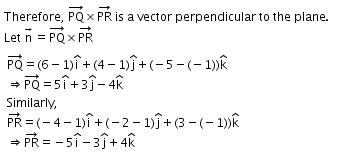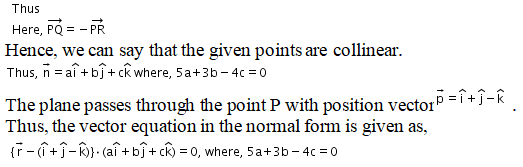Q5.

Solution:

Let us consider three points be A, B and C.

So now let A, B, C be the points with position vector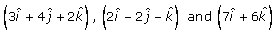.

Then,